Physic Tutorials

# Notes on magnetic field for Cambridge A level and UTME

Notes on magnetic field for Cambridge A level and UTME

Magnetic field is a region of space where a magnet or a current carrying conductor or a moving charge experience a magnetic force.

Magnetic field is directed from a North pole to a South pole.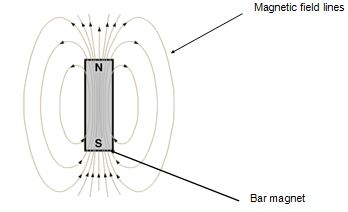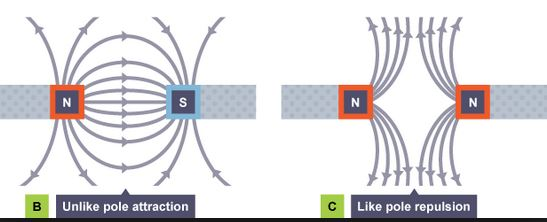A uniform field, within which the field strength is the same at all points, could be represented as parallel lines that are equally spaced as shown above. The uniform field is stronger if the lines are closer to each other.Magnetic field around a current carrying wire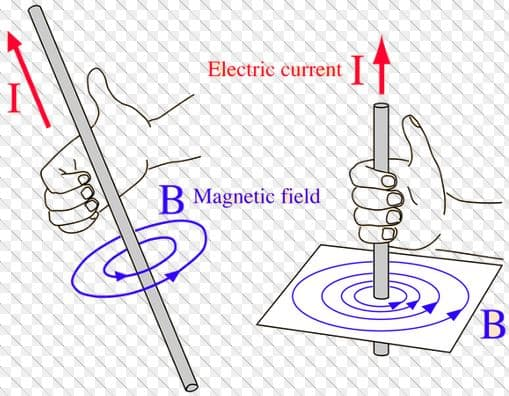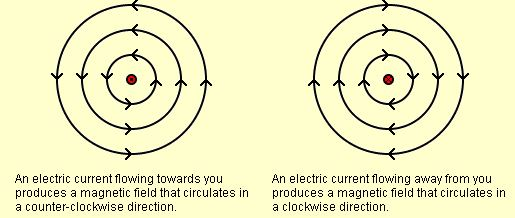When an electric current flow towards you(out of the plane paper), it produces a magnetic field that circulates in anti-clockwise direction. When electric current flow away from you(into the plane paper), it produces field that circulates in a clockwise direction.

Out of the paper is represented by a dot (.)

Into the plane paper is represented by a cross ( x)

Electromagnetism

Force on a current carrying conductorThe direction of the force can be detect using Fleming’s left hand rule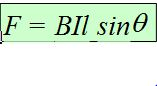• Magnetic Field, B
• Current, I
• Length of conductor in magnetic field, l
• Angle between field and current, θ

For maximum force, θ = 90

F = BIL

B is the magnetic field strength

Magnetic flux density in a magnetic field is defined as the force per unit length acting on a conductor carrying a unit current placed at right angles to the field.

Tesla: The uniform magnetic flux density which, acting normally to a long straight wire carrying a current of 1 amp, causes a force per unit length of 1 Nm-1 on the conductor

Forces between current-carrying conductors and predict the direction of the forcesWhen the currents are moving in the same direction, the forces between the current carrying conductor is attractive. When the currrents are moving in opposite direction, the forces is repulsive.

Regardless of the current, the forces on the conductor will be the same.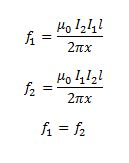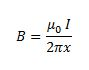x is the distance between the two parallel conductors

A moving charge in a magnetic fieldF = BQv sinθ

For a moving charge in a magnetic field…

• Magnetic Field, B
• Charge, q
• Velocity of the charge, v
• Angle between field and velocity of charge,θ

Rectangular ring in a uniform magnetic field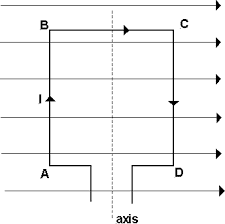F = NBIL

N is the number of turns pivoted so that it can rotate about a vertical axis

Torque = force x perpendicular distance

Electromagnetic Induction

Faraday noticed that an emf is induced in a circuit whenever there is change in magnetic flux linked with a circuit

Ø = BA

Ø is the magnetic flux and A is the area

Magnetic flux is the product of magnetic flux density and the area normal to the magnetic flux. the unit is weber

NØ  is the magnetic flux linkage

Faraday’s law state that emf induced is directly proportional to the rate of change of magnetic flux linkage.

E α dNØ/dt

Lenz’s law state that the induced emf move in such a way as to oppose the change producing it

E = -NdØ/dt

Lenz’s law is the law of conservation of energy

E = Blv

E is the emf induced

B is the magnetic field strength

l is the length

v is the velocity

Click for answers to some UTME questions on Magnetic field

### Bolarinwa Olajire

A lecturer, Educationist, PhD student at FUNAAB, and a Blogger.